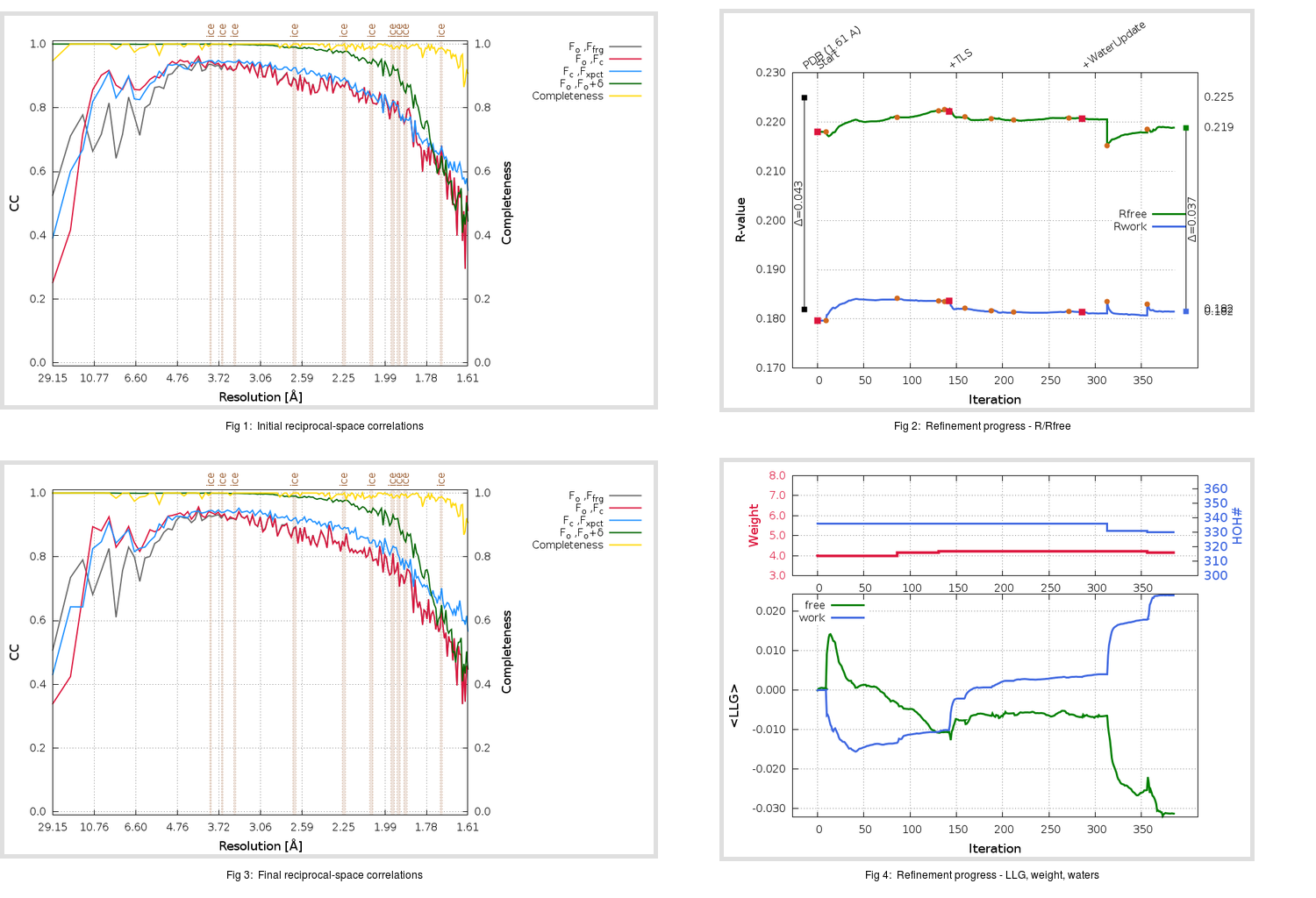Content:

```    Diffraction limits & principal axes of ellipsoid fitted to diffraction cut-off surface:
1.757         0.7099   0.0000  -0.7043       0.905 a* - 0.426 c*
1.546         0.0000   1.0000   0.0000       b*
1.653         0.7043   0.0000   0.7099       0.958 a* + 0.286 c*
```

## Deposited

` `
 Date deposited Date data collection Resolution R, Rfree 20200315 20200305 1.61 0.1800 0.2250

Molprobity (CCP4 7.0 version) summary:

```Ramachandran outliers =   0.33 %
favored =  98.34 %
Rotamer outliers      =   0.37 %
C-beta deviations     =     0
Clashscore            =   5.13
RMS(bonds)            =   0.0133
RMS(angles)           =   1.82
MolProbity score      =   1.27
Resolution            =   1.61
R-work                =   0.1800
R-free                =   0.2250
```

```Number of waters      =   336

<B> (all atoms) =   24.25 ( sd =    9.46 ) for       2795 non-hydrogen atoms
<B>   (protein) =   22.75 ( sd =    8.50 ) for       2440 non-hydrogen atoms
<B>     (water) =   34.42 ( sd =    9.47 ) for        336 non-hydrogen atoms
<B>    (others) =   32.31 ( sd =   10.61 ) for         19 non-hydrogen atoms

B min/max       (all non-hydrogen atoms) =   12.31 /   71.03
B min/max   (protein non-hydrogen atoms) =   12.31 /   71.03
B min/max     (water non-hydrogen atoms) =   13.40 /   64.56
B min/max     (other non-hydrogen atoms) =   25.24 /   42.02
```

## BUSTER (re-)refinement

` `

Molprobity (CCP4 7.0 version) summary:

```Ramachandran outliers =   0.33 %
favored =  98.68 %
Rotamer outliers      =   1.83 %
C-beta deviations     =     0
Clashscore            =   2.67
RMS(bonds)            =   0.0116
RMS(angles)           =   1.55
MolProbity score      =   1.25
Resolution            =   1.61
R-work                =   0.1815
R-free                =   0.2189
```

```Number of waters      =   330

<B> (all atoms) =   25.24 ( sd =    9.00 ) for       2789 non-hydrogen atoms
<B>   (protein) =   23.52 ( sd =    7.32 ) for       2440 non-hydrogen atoms
<B>     (water) =   37.07 ( sd =   10.48 ) for        330 non-hydrogen atoms
<B>    (others) =   34.08 ( sd =   11.08 ) for         19 non-hydrogen atoms

B min/max       (all non-hydrogen atoms) =   13.99 /   76.79
B min/max   (protein non-hydrogen atoms) =   13.99 /   63.79
B min/max     (water non-hydrogen atoms) =   14.95 /   76.79
B min/max     (other non-hydrogen atoms) =   27.50 /   44.03
```

Refinement progression:Results:

` `
 File Remark 5RF4_aB_refine.01_03_refine.pdb.gz exact refinement commands are in header 5RF4_aB_refine.01_03_refine.mtz.gz including original deposited data and several re-refinement map coefficients 5RF4_aB_refine.01_03_BUSTER_model.cif.gz including any non-standard compound restraints 5RF4_aB_refine.01_03_BUSTER_refln.cif.gz